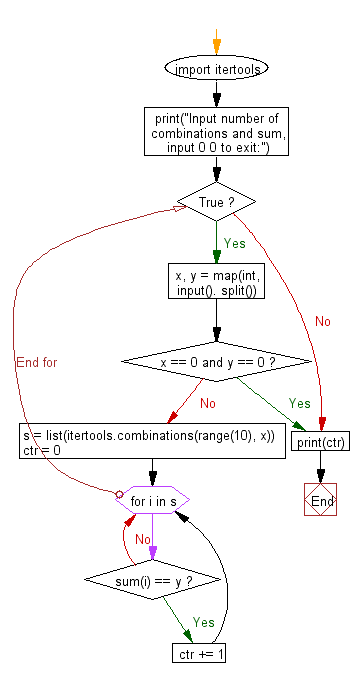﻿ Python: Reads n digits chosen from 0 to 9 and prints the number of combinations where the sum of the digits equals to another given number(s) - w3resource# Python: Reads n digits chosen from 0 to 9 and prints the number of combinations where the sum of the digits equals to another given number(s)

## Python Basic - 1: Exercise-48 with Solution

Write a Python program that reads n digits (given) chosen from 0 to 9 and prints the number of combinations where the sum of the digits equals to another given number (s). Do not use the same digits in a combination.

Input:
Two integers as number of combinations and their sum by a single space in a line. Input 0 0 to exit.

Sample Solution:

Python Code:

``````import itertools
print("Input number of combinations and sum, input 0 0 to exit:")
while True:
x, y = map(int, input(). split())
if x == 0 and y == 0:
break
s = list(itertools.combinations(range(10), x))
ctr = 0
for i in s:
if sum(i) == y:
ctr += 1

print(ctr)
``````

Sample Output:

```Input number of combinations and sum, input 0 0 to exit:
5 6
2 4
0 0
2
```

Flowchart:Python Code Editor:

Have another way to solve this solution? Contribute your code (and comments) through Disqus.

What is the difficulty level of this exercise?

Test your Programming skills with w3resource's quiz.

﻿

## Python: Tips of the Day

What is the difference between Python's list methods append and extend?

append: Appends object at the end.

```x = [1, 2, 3]
x.append([4, 5])
print (x)
```

Output:

```[1, 2, 3, [4, 5]]
```

extend: Extends list by appending elements from the iterable.

```x = [1, 2, 3]
x.extend([4, 5])
print (x)
```

Output:

```[1, 2, 3, 4, 5]
```

Ref: https://bit.ly/2AZ6ZFq